### Answers − Solving Equations 2

#### 1.   If 42 + y = 20 + 3y     solve y

i. Subtract 20 from both sides

 42 + y = 20 + 3y − 20 = − 20
 22 + y = + 3y

ii. Move y to one side. So subtract y from both sides

 22 + y = + 3y − y = − y
 22 = 2y

iii. Divide both sides by 2

 22 = 2y ÷ 2 = ÷ 2
 11 = y

iv. To verify, put the value of y = 11 back into the original equation:

 both sides are equal

#### 2.   If 6 + 4b = 15 + b     solve b

i. Subtract 6 from both sides

 6 + 4b = 15 + b − 6 = − 6
 + 4b = 9 + b

ii. Move b to one side. So subtract b from both sides

 + 4b = 9 + b − b = − b
 3b = 9

iii. Divide both sides by 3

 3b = 9 ÷ 3 = ÷ 3
 b = 3

iv. To check, put the value of b = 3 back into the original equation:

 both sides are equal

#### 3.   If 37 + t = 2(26 + 3t)     what is the value of t?

i. Multiply the brackets on RHS

 37 + t = 2(26 + 3t)
 37 + t = 52 + 6t

ii. Subtract 52 from both sides

 37 + t = 52 + 6t − 52 = − 52
 − 15 + t = 6t

iii. Move t to one side. So subtract t from both sides

 − 15 + t = 6t − t = − t
 − 15 = 5t

iv. Divide both sides by 5

 − 15 = 5t ÷ 5 = ÷ 5
 − 3 = t

v. To verify, put the value of t = −3 back into the original equation:

 both sides are equal

#### 4.   If 2(9 + y) = 4(7 + 3y)     what is the value of y?

i. Multiply the brackets on LHS and RHS

 2(9 + y) = 4(7 + 3y)
 18 + 2y = 28 + 12y

ii. Subtract 28 from both sides

 18 + 2y = 28 + 12y − 28 = − 28
 − 10 + 2y = 12y

iii. Move 2y to one side. So subtract 2y from both sides

 − 10 + 2y = 12y − 2y = − 2y
 − 10 = 10y

iv. Divide both sides by 10

 − 10 = 10y ÷ 10 = ÷ 10
 − 1 = y

iv. To check, put the value of y = −1 back into the original equation:

 both sides are equal

#### 5.   If 3(y − 120) = 5(y + 30)     what is the value of y?

i. Multiply the brackets on LHS and RHS

 3(y − 120) = 5(y + 30)
 3y − 360 = 5y + 150

ii. Subtract 150 from both sides

 3y − 360 = 5y + 150 − 150 = − 150
 3y − 510 = 5y

iii. Move 3y to one side. So subtract 3y from both sides

 3y − 510 = 5y − 3y = − 3y
 − 510 = 2y

iv. Divide both sides by 2

 − 510 = 2y ÷ 2 = ÷ 2
 − 255 = y

v. To verify, put the value of y = −255 back into the original equation:

 both sides are equal

#### what is the value of b?

i. Multiply both sides by 7= 7 × 7 = × 7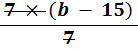= 49
 b − 15 = 49

ii. Add 15 to both sides

 b − 15 = 49 + 15 = +15
 b = 64

iii. To check, put the value of b = 64 back into the original equation:#### what is the value of y?

i. Multiply both sides by 12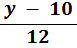= −5 × 12 = × 12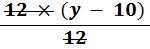= −60
 y − 10 = −60

ii. Add 10 to both sides

 y − 10 = −60 + 10 = +10
 y = −50

iii. To verify, put the value of y = −50 back into the original equation:#### what is the value of b?

i. Multiply both sides by 4= 3(b + 10) × 4 = × 4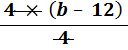= 12(b + 10)
 b − 12 = 12b + 120

ii. Subtract 120 from both sides

 b − 12 = 12b + 120 − 120 = − 120
 b − 132 = 12b

iii. Subtract b from both sides

 b − 132 = 12b − b = −b
 − 132 = 11b

iv. Divide both sides by 11

 − 132 = 11b ÷ 11 = ÷11
 −12 = b

v. To check, put the value of b = −12 back into the original equation:

 both sides are equal

#### what is the value of b?

i. Multiply both sides by 7

 6(17 + b) =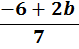× 7 = × 7
 42(17 + b) =714 + 42b = −6 + 2b

ii. Subtract 714 from both sides

 714 + 42b = −6 + 2b − 714 = −714
 42b = − 720 + 2b

iii. Subtract 2b from both sides

 42b = − 720 + 2b − 2b = − 2b
 40b = − 720

iv. Divide both sides by 40

 40b = − 720 ÷ 40 = ÷ 40
 b = −18

v. To verify, put the value of b = −18 back into the original equation:

 both sides are equalback to: